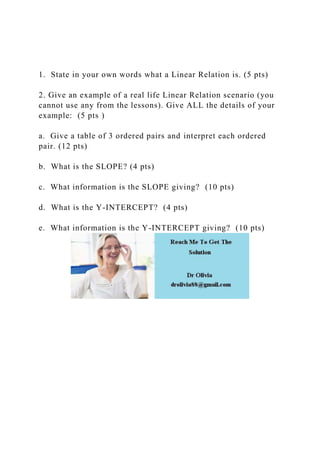Successfully reported this slideshow.

# 1.  State in your own words what a Linear Relation is. (5 pts).docx×

# 1.  State in your own words what a Linear Relation is. (5 pts).docx

1.  State in your own words what a Linear Relation is. (5 pts)

2. Give an example of a real life Linear Relation scenario (you cannot use any from the lessons). Give ALL the details of your example:  (5 pts )

a.  Give a table of 3 ordered pairs and interpret each ordered pair. (12 pts)

b.  What is the SLOPE? (4 pts)

c.  What information is the SLOPE giving?  (10 pts)

d.  What is the Y-INTERCEPT?  (4 pts)

e.  What information is the Y-INTERCEPT giving?  (10 pts)
.

1.  State in your own words what a Linear Relation is. (5 pts)

2. Give an example of a real life Linear Relation scenario (you cannot use any from the lessons). Give ALL the details of your example:  (5 pts )

a.  Give a table of 3 ordered pairs and interpret each ordered pair. (12 pts)

b.  What is the SLOPE? (4 pts)

c.  What information is the SLOPE giving?  (10 pts)

d.  What is the Y-INTERCEPT?  (4 pts)

e.  What information is the Y-INTERCEPT giving?  (10 pts)
.